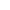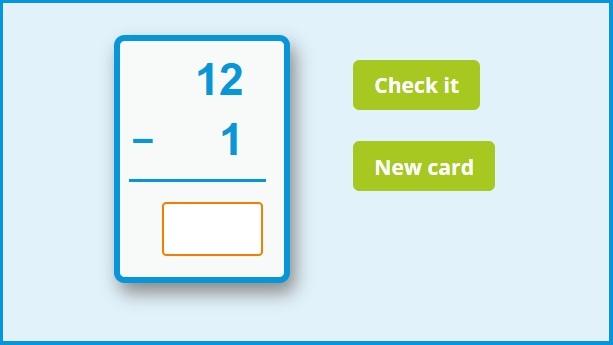﻿ Subtraction flash cards online FREE

Page No. 1084

# Subtraction flash cards online

Enjoy math subtraction flash cards online FREE. Math subtraction cards to 20. You can choose the maximum sum of our free online subtraction flash cards: to 20, 30, 50 or 100. Learn subtraction fast with interactive subtraction flash cards. Subtraction task cards. Free subtraction flash cards are perfect for improving subtraction skills at any age. Math flash cards subtraction. Math subtraction flash cards 0-20 online. Subtraction facts to 20 flashcards. Free online subtraction flash cards to 20, 30, 50 and 100.Insert the result of the subtraction fact to the empty box.

## Math subtraction flash cards online FREEOnline subtraction flash cards help kids to master subtraction up to 100. Insert the result of the subtraction task to the empty box. Press "Check it" to find out if the outcome of subtraction is correct. If the result is OK, the box will be green. If your answer isn´t correct, the box will be red and you will see the correct answer.

Press the button "New card" and a new online subtraction flash card will appear on the screen.

#### Math flash cards online

Try also these online flash cards:

## Online subtraction flash cards to 20, 30, 50 and 100

Play our online subtraction flash cards game. You can choose the sum of these free subtraction flash cards: less than 20, 30, 50 or 100. Subtraction task cards are perfect for quick practicing of subtraction facts to 100.

Online subtraction flashcards complement well with these colorful quality cards that range from 0 - 12.

### Math subtraction cards

These subtraction cards are fantastic alongside other subtractions resources. Here are a few you might be interested in:

### How to use online subtraction flash cards

Subtraction is a mathematical process where one number is taken away from another. For example, 4 subtracted from 10 is 6. These subtraction cards teach subtract from 100 down. Online subtraction flashcards are great for reinforcing your pupils' subtraction skills or for testing children's knowledge. Check free subtraction worksheets here.

Category: